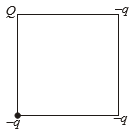If E be the electric field inside a parallel plate capacitor due to Q and -Q charges on the two plates, then electrostatic force on plate having charge -Q due to the plate having charge +Q will be

(1) -QE

(2) $\frac{-QE}{2}$

(3) QE

(4) $\frac{-QE}{4}$

Concept Questions :-

Capacitance
High Yielding Test Series + Question Bank - NEET 2020

Difficulty Level:

If W be the amount of heat produced in the process of charging an uncharged capacitor then the amount of energy stored in it is

(1) 2W

(2) $\frac{W}{2}$

(3) W

(4) zero

Concept Questions :-

Energy stored in capacitor
High Yielding Test Series + Question Bank - NEET 2020

Difficulty Level:

A metallic sphere of capacitance ${C}_{1}$, charged to electric potential ${V}_{1}$ is connected by a metal wire to another metallic sphere of capacitance ${C}_{2}$ charged to electric potential ${V}_{2}$. The amount of heat produced in the connecting wire during the process is

(1)$\frac{{C}_{1}{C}_{2}}{2\left({C}_{1}+{C}_{2}\right)}{\left({V}_{1}+{V}_{2}\right)}^{2}$

(2) $\frac{{C}_{1}{C}_{2}}{2\left({C}_{1}+{C}_{2}\right)}{\left({V}_{1}-{V}_{2}\right)}^{2}$

(3) $\frac{{C}_{1}{C}_{2}}{{C}_{1}+{C}_{2}}{\left({V}_{1}-{V}_{2}\right)}^{2}$

(4) zero

Concept Questions :-

Energy stored in capacitor
High Yielding Test Series + Question Bank - NEET 2020

Difficulty Level:

The electric potential at the surface of a charged solid sphere of insulator is 20V. The value of electric potential at its centre will be

1.  30V

2.  20V

3.  40V

4.  Zero

Concept Questions :-

Electric potential
High Yielding Test Series + Question Bank - NEET 2020

Difficulty Level:

In the situation shown in the figure, what should be the relation between Q and q so that electric potential at the centre of the square is zero1.  Q = q

2.  Q = 3q

3.  Q = 2q

4.  Q = -3q

Concept Questions :-

Electric potential
High Yielding Test Series + Question Bank - NEET 2020

Difficulty Level:

The capacitance of a parallel plate capacitor is C. If a dielectric slab of thickness equal to one-fourth of the plate separation and dielectric constant K is inserted between the plates, then new capacitance become

1. $\frac{KC}{2\left(K+1\right)}$

2. $\frac{2KC}{K+1}$

3. $\frac{5KC}{4K+1}$

4. $\frac{4KC}{3K+1}$

Concept Questions :-

Dielectrics
High Yielding Test Series + Question Bank - NEET 2020

Difficulty Level:

The electric potential at a point in space due to charge Q is Q V. The value of an electric field at that point will be

1.  $\frac{\mathrm{Q}}{9}×{10}^{12}\frac{\mathrm{N}}{\mathrm{C}}$

2.  $\frac{\mathrm{Q}}{9}×{10}^{15}\frac{\mathrm{N}}{\mathrm{C}}$

3.  $\frac{\mathrm{Q}}{9}×{10}^{9}\frac{\mathrm{N}}{\mathrm{C}}$

4.

Concept Questions :-

Relation between field and potential
High Yielding Test Series + Question Bank - NEET 2020

Difficulty Level:

The electric potential at a point at distance 'r' from a short dipole is proportional to

(1) ${r}^{2}$

(2) ${\mathrm{r}}^{-1}$

(3) ${r}^{-2}$

(4) ${r}^{1}$

Concept Questions :-

Electric potential
High Yielding Test Series + Question Bank - NEET 2020

Difficulty Level:

A hollow charged metal spherical shell has radius R. If the potential difference between its surface and a point at a distance 3R from the center is V, then the value of electric field intensity at a point at distance 4R from the center is

1.  $\frac{3\mathrm{V}}{19\mathrm{R}}$

2.  $\frac{\mathrm{V}}{6\mathrm{R}}$

3.  $\frac{3\mathrm{V}}{32\mathrm{R}}$

4.  $\frac{3\mathrm{V}}{16\mathrm{R}}$

Concept Questions :-

Relation between field and potential
High Yielding Test Series + Question Bank - NEET 2020

Difficulty Level:

A metallic sphere is given some charge. Electric energy is stored

(1) Only inside the sphere

(2) Only outside the sphere

(3) Both inside and outside

(4) Neither inside nor outside

Concept Questions :-

Electric potential energy